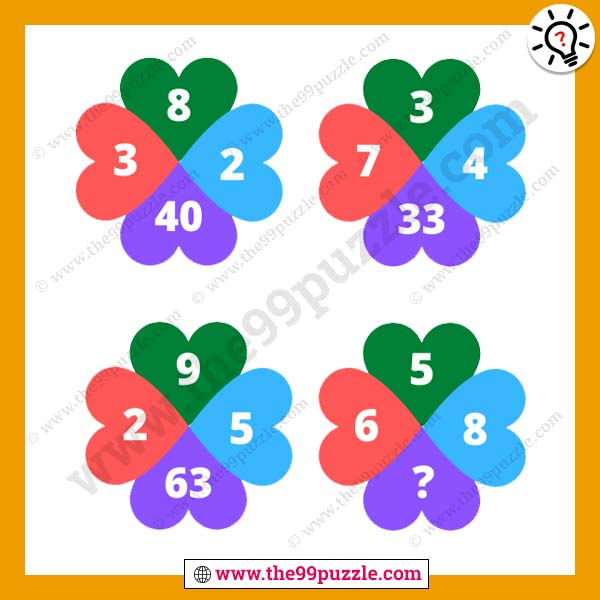# Fun and reasoning number puzzle with answer – Puzz256

Can you solve the fun and reasoning number puzzle? This puzzle can twist your logical brain when you are trying to solve this math riddle. Here you see four-figure, each figure has math logic. You have to crack the math logic and replace the question mark. Here you will find many logical reasoning missing number puzzles for students. If you are good at puzzles you can find the value of these brain teaser letter puzzles.###### Explanation:

1st Figure (Top Left) = 8×(3+2) = 40

2nd Figure (Top Right) = 3×(7+4) = 33

3rd Figure (Bottom Left) = 9×(2+5) = 63

4th Figure (Bottom Right) = 5×(6+8) = 70